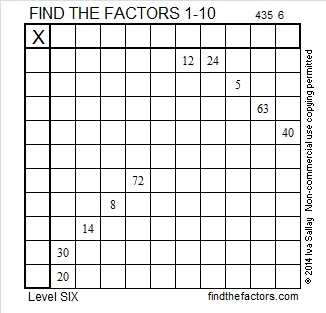# 225 and Level 6

• 225 is a composite number and a perfect square.
• Prime factorization: 225 = 3 x 3 x 5 x 5, which can be written 225 = (3^2) x (5^2)
• The exponents in the prime factorization are 2 and 2. Adding one to each and multiplying we get (2 + 1)(2 + 1) = 3 x 3 = 9. Therefore 225 has 9 factors.
• Factors of 225: 1, 3, 5, 9, 15, 25, 45, 75, 225
• Factor pairs: 225 = 1 x 225, 3 x 75, 5 x 45, 9 x 25, or 15 x 15
• √225 = 15Excel file of puzzles and previous week’s factor solutions: 10 Factors 2014-09-01This site uses Akismet to reduce spam. Learn how your comment data is processed.